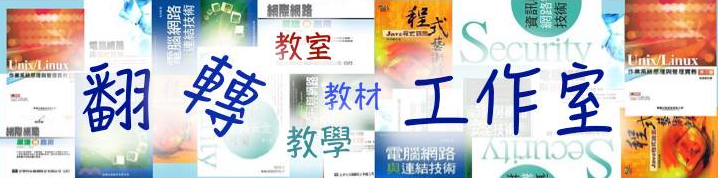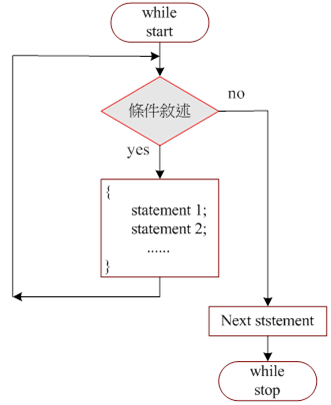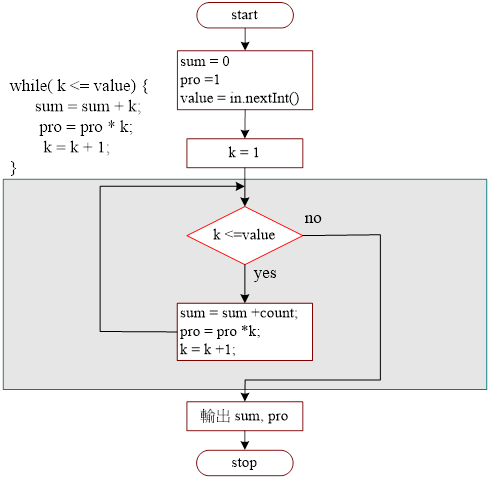Java 程式設計(一)  ：第 五章 重複性流程5-3 while 迴圈敘述

• 5-3-1 while 敘述的流程

• 5-3-2 範例研討：累加/累乘程式

• 5-3-3 範例研討：製作電子算盤

• 5-3-4 自我挑戰：超商收銀機

5-3-1 while 敘述的流程

5 -4  while 敘述的運作流程圖，首先判斷條件是否成立，成立（yes）則執行 while 迴圈實體，否則（no）不執行 while 迴圈，並離開此 while 敘述；當條件成立並執行迴圈實體之後，再測試條件是否成立，如成立則繼續執行迴圈，否則跳離迴圈。5-4 While 敘述的流程圖

 語法： 範例： while (條件判斷) { statement 1; statement 2; …. } k=1; while(k <= value) {      sum = sum + k;      pro = pro * k;      k =  k +1; }

A）程式功能：Ex5_3.java

 請輸入一個整數 =>20 1+2+3+4+...+20 = 210 1*2*3*... *20 = 2432902008176640000

B）製作技巧研討：5-5 while 敘述製作累加/累乘程式

C）程式範例：

 01 02 03 04 05 06 07 08 09 10 11 12 13 14 15 16 17 18 19 //Ex5_3.java   import java.util.*; public class Ex5_3 {      public static void main(String args[]) {           Scanner keyin = new Scanner(System.in);           long sum=0, pro=1, value, k;           System.out.printf("請輸入一個整數 =>");           value = keyin.nextInt();           k=1;           while(k <= value) {              sum = sum + k;              pro = pro * k;              k =  k +1;           }           System.out.printf("1+2+3+4+...+%d = %d\n", value, sum);           System.out.printf("1*2*3*... *%d = %d\n", value, pro);      } }

D）程式重點說明：

• 7 long sum=0, pro=1;。分別設定累加與累乘之累積器的初始值。

• 10 k=1;。初始值設定，使用於 while 敘述的條件判斷式。

• 11~15 while (k <=value) { …..}While 敘述句，判斷 k <= value 條件成立的話，則執行迴圈內敘述區塊；否則結束 while 敘述。

• 14 k = k+1;。條件值增量，如果沒有此敘述，while 迴圈將永遠做不完。

A）程式功能：Ex5_4.java

 歡迎光臨 == 春嬌柑仔店 ==   已購買 0 請輸入產品價格 (0 結束) =>50 已購買 50 請輸入產品價格 (0 結束) =>76 已購買 126 請輸入產品價格 (0 結束) =>230 已購買 356 請輸入產品價格 (0 結束) =>0 人客您總共買 356 元 謝謝!! 愛過來ㄜ

B）製作技巧研討：

 導入輸入套件； 宣告相關變數，並設定適當初值； 顯示目前購買小計（total），並要求輸入下一個產品價格； 讀入產品價格（cost）； while (cost > 0 ) { 累計購買小計（total = total + cost）； 顯示目前購買小計（total），並要求輸入下一個產品價格； 讀入產品價格（cost）； } 顯示客戶總購買金額；

C）程式範例：

 01 02 03 04 05 06 07 08 09 10 11 12 13 14 15 16 17 18 19 //Ex5_4.java   import java.util.*; public class Ex5_4 {    public static void main(String args[]) {       Scanner keyin = new Scanner(System.in);       int total=0, cost;       System.out.printf("歡迎光臨 == 春嬌柑仔店 ==\n\n");       System.out.printf("已購買 %d 請輸入產品價格 (0 結束) =>", total);       cost = keyin.nextInt();       while (cost > 0) {           total = total + cost;           System.out.printf("已購買 %d 請輸入產品價格 (0 結束) =>", total);           cost = keyin.nextInt();       }       System.out.printf("人客您總共買 %d 元 謝謝!! 愛過來ㄜ\n", total);    } }

A 程式功能：PM5_2.java

 *** 歡迎光臨  春嬌超商 *** 累計 0.0 請輸入貨品單價 (0 結束) =>56         請輸入購買數量 =>2 累計 112.0 請輸入貨品單價 (0 結束) =>67         請輸入購買數量 =>4 累計 380.0 請輸入貨品單價 (0 結束) =>238         請輸入購買數量 =>2 累計 856.0 請輸入貨品單價 (0 結束) =>0 總計 => 856.0

B）製作技巧提示：

 … 設定購買總額初值（total =0） 顯示目前購買小計（total），並要求輸入下一個產品價格； 讀入產品價格（cost）； while (cost > 0 ) { 顯示輸入購買數量，並讀取（number）; 累計購買小計（total = total + cost*number）； 顯示目前購買小計（total），並要求輸入下一個產品價格； 讀入產品價格（cost）； } 顯示客戶總購買金額；

Java 程式設計(一) 含程式邏輯